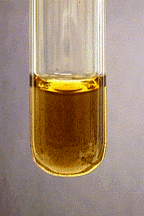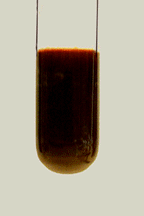# Characteristic Reactions of Manganese Ions (Mn²⁺)

$$\newcommand{\vecs}{\overset { \rightharpoonup} {\mathbf{#1}} }$$ $$\newcommand{\vecd}{\overset{-\!-\!\rightharpoonup}{\vphantom{a}\smash {#1}}}$$$$\newcommand{\id}{\mathrm{id}}$$ $$\newcommand{\Span}{\mathrm{span}}$$ $$\newcommand{\kernel}{\mathrm{null}\,}$$ $$\newcommand{\range}{\mathrm{range}\,}$$ $$\newcommand{\RealPart}{\mathrm{Re}}$$ $$\newcommand{\ImaginaryPart}{\mathrm{Im}}$$ $$\newcommand{\Argument}{\mathrm{Arg}}$$ $$\newcommand{\norm}{\| #1 \|}$$ $$\newcommand{\inner}{\langle #1, #2 \rangle}$$ $$\newcommand{\Span}{\mathrm{span}}$$ $$\newcommand{\id}{\mathrm{id}}$$ $$\newcommand{\Span}{\mathrm{span}}$$ $$\newcommand{\kernel}{\mathrm{null}\,}$$ $$\newcommand{\range}{\mathrm{range}\,}$$ $$\newcommand{\RealPart}{\mathrm{Re}}$$ $$\newcommand{\ImaginaryPart}{\mathrm{Im}}$$ $$\newcommand{\Argument}{\mathrm{Arg}}$$ $$\newcommand{\norm}{\| #1 \|}$$ $$\newcommand{\inner}{\langle #1, #2 \rangle}$$ $$\newcommand{\Span}{\mathrm{span}}$$$$\newcommand{\AA}{\unicode[.8,0]{x212B}}$$

• Most common oxidation states: +2, +7; +3, +4, and +6 also exist.
• M.P. 1244º
• B.P. 1962º
• Density 7.43 g/cm3
• Characteristics: Manganese is a gray or reddish-white metal. Very hard and brittle. Very similar to iron in activity. Dissolves readily in dilute acids.

## Characteristic Reactions of Mn²⁺

### Aqueous Ammonia

Addition of aqueous ammonia precipitates white $$\ce{Mn(OH)2}$$:

$\ce{Mn^{2+}(aq) + 2NH3(aq) + 2H2O(l) <=> Mn(OH)2(s) + 2NH4^{+}(aq)} \nonumber$The precipitate does not dissolve in excess ammonia, but does dissolve in solutions containing ammonium salts. The precipitate is easily oxidized by atmospheric oxygen to form Mn(III) or Mn(IV), which turns the precipitate a brownish color.

### Sodium Hydroxide

Sodium hydroxide precipitates manganese(II) hydroxide:

$\ce{Mn^{2+}(aq) + 2OH^{-}(aq) <=> Mn(OH)2(s) } \nonumber$### Hydrogen Peroxide

In basic solutions, $$\ce{H2O2}$$ oxidizes Mn(II) to Mn(IV), giving a brown precipitate:

$\ce{Mn(OH)2(s) + H2O2(aq) -> MnO2(s) + 2H2O(l)} \nonumber$$$\ce{MnO2}$$ is generally insoluble in acids, but does react with hot concentrated hydrochloric acid to release chlorine gas. In acid solution, $$\ce{H2O2}$$ becomes a reducing agent, and the $$\ce{MnO2}$$ will dissolve:

$\ce{MnO2(s) + H2O2(aq) + 2H^{+}(aq) -> Mn^{2+}(aq) + O2(g) + 2H2O(l)} \nonumber$### Sodium Bismuthate

Solid sodium bismuthate oxidizes $$\ce{Mn^{2+}}$$ to purple $$\ce{MnO4^{-}}$$ without heating. With heating, the product is $$\ce{MnO2}$$.

$\ce{2Mn^{2+}(aq) + 5BiO3^{-}(aq) + 14H^{+}(aq) -> 2MnO4^{-}(aq) + 5BiO^{+}(aq) + 7H2O(l)} \nonumber$### No Reaction

$$\ce{Cl^{-}}$$, $$\ce{SO4^{2-}}$$

This page titled Characteristic Reactions of Manganese Ions (Mn²⁺) is shared under a CC BY-NC-SA 4.0 license and was authored, remixed, and/or curated by James P. Birk.